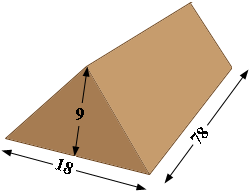SEARCH HOMEMath Central Quandaries & QueriesQuestion from Tim: I have a pile of compost that is 78 feet long by 18 feet wide at base by 9 feet tall to a point. At the width it looks like a triangle I'm looking for the square yards ThanksHi Tim,

I drew what I think is the shape of your compost pile (not to scale).The volume is the area of the triangular face times the length. Since the dimensions are in fee this will give the volume in cubic feet. To obtain the volume in cubic yards I would first convert the dimensions to yards. There are 3 feet in a yard so pile is 78/3 yards long by 18/3 = 6 yards wide by 9/3 = 3 yards high.

The area of a triangle s one half the base times the height and hence the volume of your compost pile is

1/2 × 6 × 3 × 78/3 = 234 cubic yards.

PennyMath Central is supported by the University of Regina and The Pacific Institute for the Mathematical Sciences.